Reach Us+44-7480-724769
Chief Factors of Lie Algebras | OMICS International
Journal of Generalized Lie Theory and Applications
All submissions of the EM system will be redirected to Online Manuscript Submission System. Authors are requested to submit articles directly to Online Manuscript Submission System of respective journal.

Chief Factors of Lie Algebras

Towers DA*

Department of Mathematics and Statistics, Lancaster University, LA1 4YF Lancaster, UK

Corresponding Author:
Towers DA
Department of Mathematics and Statistics
Lancaster University, LA1 4YF Lancaster, UK
Tel: +44152465201
E-mail: [email protected]

Received Date: December 23, 2015; Accepted Date: January 05, 2016; Published Date: January 07, 2016

Citation: Towers DA (2016) Chief Factors of Lie Algebras. J Generalized Lie Theory Appl S2:e002. doi:10.4172/1736-4337.S2-e002

Copyright: © 2016 Towers DA. This is an open-access article distributed under the terms of the Creative Commons Attribution License, which permits unrestricted use, distribution, and reproduction in any medium, provided the original author and source are credited.

Visit for more related articles at Journal of Generalized Lie Theory and Applications

Abstract

In group theory the chief factors allow a group to be studied by its representation theory on particularly natural irreducible modules. It is to be expected, therefore, that they will play an important role in the study of Lie algebras. In this article we survey a few of their properties.

Keywords

L-Algebras; L-Equivalence; c-factor; m-factor; cc-type

Introduction

Throughout L will denote a finite-dimensional Lie algebra over a field F. We call a subalgebra I a subideal of a Lie algebra L if there is a chain of subalgebras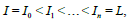where Ij is an ideal of Ij+1 for each 0 ≤ j ≤ n −1 .

Put L1 = L, Lk+1 = [Lk, L] for k ≥ 1. These are the terms of the lower central series for L. We say that L has nilpotency class n if Ln ≠ 0 but Ln+1 = 0 . Let U be a subalgebra of L. If F has characteristic p>0 we call U nilregular if the nilradical of U, N(U), has nilpotency class less than p − 1. If F has characteristic zero we regard every subalgebra of L as being nilregular. We say that U is characteristic in L if it is invariant under all derivations of L. Nilregular ideals of L have the property that their nilradicals are characteristic in L. Details of the results in this section can be found in studies of Towers .

Theorem 1.1.

(i) If I is a nilregular ideal of L then N(I ) ⊆ N(L).

(ii) If I is a nilregular subideal of L and every subideal of L containing I is nilregular, then N(I ) ⊆ N(L).

This result was proved by Schenkman  for fields of characteristic zero; in characteristic p it follows from a more recent result of Maksimenko . Similarly, we will call the subalgebra U solregular if the underlying field F has characteristic zero, or if it has characteristic p and the (solvable) radical of U, R(U), has derived length less than log2p. Then we have the following corresponding theorem, which uses a result of Petravchuk .

Theorem 1.2.

(i) If I is a solregular ideal of L then R(I ) ⊆ R(L).

(ii) If I is a solregular subideal of L and every subideal of L containing I is solregular, then R(I ) ⊆ R(L) .

These enable us to determine what the minimal ideals of L look like.

Theorem 1.3. Let L be a Lie algebra over a field F, and let I be a minimal non-abelian ideal of L. Then either

(i) I is simple or

(ii) F has characteristic p, N(I) has nilpotency class greater than or equal to p − 1, and R(I) has derived length greater than or equal to log2p.

As a result of the above we will call the subalgebra U regular if it is either nilregular or solregular; otherwise we say that it is irregular. Then we have the following corollary.

Corollary 1.4. Let L be a Lie algebra over a field F. Then every minimal ideal of L is abelian, simple or irregular.

Block’s Theorem on differentiably simple rings  describes the irregular minimal ideals as follows.

Theorem 1.5. Let L be a Lie algebra over a field of characteristic p>0 and let I be an irregular minimal ideal of L. Then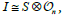where S is simple and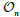is the truncated polynomial algebra in n indeterminates. Moreover, N(I) has nilpotency class p − 1 and R(I) has derived length [log2p].

Primitive Lie algebras

Next we introduce the concept of a primitive Lie algebra. Details of the results in this section can be found in literature of Towers . A word of warning - this terminology has been used for a different concept elsewhere. If U is a subalgebra of L we define UL, the core (with respect to L) of U to be the largest ideal of L contained in U. We say that U is core-free in L if UL = 0. We shall call L primitive if it has a core-free maximal subalgebra. The centraliser of U in L is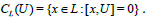There are three types of primitive Lie algebra: primitive of type 1 if it has a unique minimal ideal that is abelian; primitive of type 2 if it has a unique minimal ideal that is non-abelian; and primitive of type 3 if it has precisely two distinct minimal ideals each of which is non-abelian.

Of course, primitive Lie algebras of types 2 and 3 are semisimple, and those of types 1 and 2 are monolithic. (A Lie algebra L is called monolithic if it has a unique minimal ideal W, the monolith of L.)

Example 2.1. Examples of each type are easy to find.

Clearly every primitive solvable Lie algebra is of type 1. Every simple Lie algebra is primitive of type 2.

If S is a simple Lie algebra then L = S ⊕ S is primitive of type 3 with core-free maximal subalgebra D = {s + s : s∈S} the diagonal subalgebra of L.

Let M be a maximal subalgebra of L. Then M/ML is a core-free maximal subalgebra of L/ML We say that M is

1. a maximal subalgebra of type 1 if L/ML is primitive of type 1;

2. a maximal subalgebra of type 2 if L/ML is primitive of type 2; and

3. a maximal subalgebra of type 3 if L/ML is primitive of type 3.

We say that an ideal A is complemented in L if there is a subalgebra U of L such that L = A + U and A ∩ U = 0. For primitive solvable Lie algebras we have the following analogue of Galois’ Theorem for groups.

Theorem 2.2.

1. If L is a solvable primitive Lie algebra then all core-free maximal subalgebras are conjugate.

2. If A is a self-centralising minimal ideal of a solvable Lie algebra L, then L is primitive, A is complemented in L, and all complements are conjugate.

The Frattini ideal of L, φ(L), is the core of intersection of the maximal subalgebras of L. We say that L is φ-free if φ(L) = 0. Then we have the following characterisation of primitive Lie algebras of type 1.

Theorem 2.3. Let L be a Lie algebra over a field F.

1. L is primitive of type 1 if and only if L is monolithic, with abelian monolith W, and φ-free.

If F has characteristic zero, then L is primitive of type 1 if and only if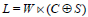(semi-direct sum), where W is the abelian monolith of L, C is an abelian subalgebra of L, every element of which acts semisimply on W, and S is a Levi subalgebra of L.

If L is solvable, then L is primitive if and only if it has a selfcentralising minimal ideal A.

For type 2 we have

Theorem 2.4.

L is primitive of type 2 if and only if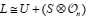, where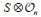is an ideal of L and S is simple.

If F has characteristic zero, then L is primitive of type 2 if and only if L is simple.

L is primitive of type 2 if and only if there is a primitive Lie algebra X of type 3 such that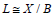for a minimal ideal B of L.

For type 3 we have

Theorem 2.5.

L is primitive of type 3 if and only if L has two distinct minimal ideals B1 and B1 with a common complement and such that the factor algebras L/B1 are primitive of type 2 for i = 1, 2. Moreover, B1 and B1 are both isomorphic to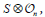where S is simple.

If F has characteristic zero, then L is primitive of type 3 if and only if L = S ⊕ S , where S is simple.

Chief Factors

The factor algebra A/B is called a chief factor of L if B is an ideal of L and A/B is a minimal ideal of L/B. So chief factorsare as described in Corollary 1.4 and Theorem 1.5. We can identify different types of chief factor; details for this section can be found in studies of Towers . A chief factor A/B is called Frattini if A / B ⊆φ (L / B). This concept was first introduced in literature of Towers .

If there is a subalgebra, M such that L = A + M and B ⊆ A∩M, we say that A/B is a supplemented chief factor of L and that M is a supplement of A/B in L Also, if A/B is a non-Frattini chief factor of L, then A/B is supplemented by a maximal subalgebra M of L

If A/B is a chief factor of L supplemented by a subalgebra M of L, and A∩M = B then we say that A/B is complemented chief factor of L, and M is a complement of A/B in L. When L is solvable, it is easy to see that a chief factor is Frattini if and only if it is not complemented. Then we have the following generalisation of the Jordan-Hölder Theorem.

Theorem 3.1. Let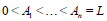(1)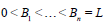(2)

be chief series for the Lie algebra L. Then there is a bijection between the chief factors of these two series such that corresponding factors are isomorphic as L-modules and such that the Frattini chief factors in the two series correspond.

The number of Frattini chief factors or of chief factors which are complemented by a maximal subalgebra of a finite-dimensional Lie algebra L is the same in every chief series for L. However, this is not the case for the number of chief factors which are simply complemented in L; in framework of Towers  we determine the possible variation in that number.

Note that if L is a primitive Lie algebra of type 3, its two minimal ideals are not L-isomorphic, so we introduce the following concept. We say that two chief factors of L are L-connected if either they are L-isomorphic, or there exists an epimorphic image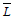of L which is primitive of type 3 and whose minimal ideals are L-isomorphic, respectively, to the given factors. (It is clear that, if two chief factors of L are L-connected and are not L-isomorphic, then they are nonabelian and there is a single epimorphic image of L which is primitive of type 3 and which connects them.) Then, as we would hope,

Theorem 3.2. The relation ‘is L-connected to’ is an equivalence relation on the set of chief factors.

Let A/B be a supplemented chief factor of L and put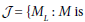a maximal subalgebra of L supplementing a chief factor L-connected to A/B}. Let R = ∩{N : N ∈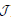} and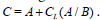Then we call C/R the crown of L associated with A/B. This object gives much information about the supplemented chief factors of L.

Theorem 3.3. Let C/R be the crown associated with the supplemented chief factor A/B of L. Then C/R = Soc(L/R). Furthermore

(i) every minimal ideal of L/R is a supplemented chief factor of L which is L-connected to A/B, and

(ii) no supplemented chief factor of L above C or below R is L-connected to A/B.

In other words, there are r ideals A1,…, Ar of L such that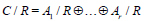where Ai/R is a supplemented chief factor of L which is L-connected to A/B for i = 1,…, r and r is the number of supplemented chief factors of L which are L-connected to A/B in each chief series for L. Moreover, φ (L / R) = 0 .

Corollary 3.4. Two supplemented chief factors of L define the same crown if and only if they are L-connected.

Theorem 3.5. Let L be a solvable Lie algebra, and let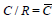be the crown associated with a supplemented chief factor of L. Then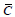is complemented in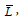and any two complements are conjugate by an automorphism of the form 1 + ad a for some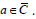Finally, in , Barnes determined for a solvable Lie algebra which irreducible L-modules A have the property that H1(L, A) = 0.

Theorem 3.6. Let L be a solvable Lie algebra and let A be an irreducible L-module. Then H1(L, A) = 0 if and only if L has no complemented chief factor isomrphic to A.

Covering and Avoidance

The subalgebra U avoids the factor algebra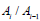if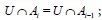likewise, U covers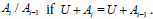We say that U has the covering and avoidance property of L if U either covers or avoids every chief factor of L. We also say that U is a CAP-subalgebra of L. Then these subalgebras give characterisations of solvable and supersolvable Lie algebras; details can be found in studies of Towers .

There are a number of ways in which CAP-subalgebras arise. For a subalgebra B of L we denote by [B : L] the set of all subalgebras S of L with B ⊆ S ⊆ L , and by [B : L]max the set of maximal subalgebras in [B : L]; that is, the set of maximal subalgebras of L containing B. We define the set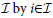if and only if 1 / i i A A− is not a Frattini chief factor of L. For each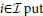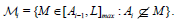Then U is a prefrattini subalgebra of L if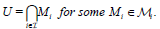It was shown in  that, when L is solvable, this definition does not depend on the choice of chief series, and that the prefrattini subalgebras of L cover the Frattini chief factors and avoid the rest; that is, they are CAP-subalgebras of L.

Further examples were given by Stitzinger , where he proved the following result  for definitions of the terminology used).

Theorem 4.1. (, Theorem 2) Let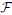be a saturated formation of solvable Lie algebras, and let U be an-normaliser of L. Then U covers every-central chief factor of L and avoids every-eccentric chief factor of L.

The chief factor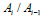is called central if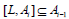and eccentric otherwise. A particular case of the above result is the following theorem, due to Hallahan and Overbeck.

Theorem 4.2. (, Theorem 1) Let L be a metanilpotent Lie algebra. Then C is a Cartan subalgebra of L if and only if it covers the central chief factors and avoids the eccentric ones.

A subalgebra U of L will be called ideally embedded in L if IL(U) contains a Cartan subalgebra of L, where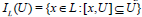is the idealiser of U in L. Clearly, any subalgebra containing a Cartan subalgebra of L and any ideal of L is ideally embedded in L. Then we have the following extension of Theorem 4.2.

Theorem 4.3. Let L be a metanilpotent Lie algebra and let U be ideally embedded in L. Then U is a CAP-subalgebra of L.

Corollary 4.4. Let L be any solvable Lie algebra and let U be an ideally embedded subalgebra of L with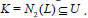Then U is a CAPsubalgebra of L.

Another set of examples of CAP-subalgebras, which don’t require L to be solvable, is given by the next result.

Theorem 4.5. Let L be any Lie algebra, let U be a supplement to an ideal B in L, and suppose that Bk ⊆U for some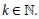Then U is a CAPsubalgebra of L.

We can calculate the dimension of CAP-subalgebras in terms of the chief factors that they cover.

Lemma 4.6. Let U be a CAP-subalgebra of L,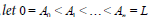be a chief series for L and let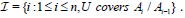Then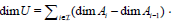We have the following characterisations of solvable and supersolvable Lie algebras.

Theorem 4.7. Every one-dimensional subalgebra of L is a CAPsubalgebra of L if and only if L is supersolvable.

Theorem 4.8. Let L be a Lie algebra over any field F. Then L is solvable if and only if all of its maximal subalgebras are CAP-subalgebras.

Theorem 4.9. Let L be a Lie algebra over a field F which has characteristic zero, or is algebraically closed field and of characteristic greater than 5. Then L is solvable if and only if there is a maximal subalgebra M of L such that M is a solvable CAP-subalgebra of L.

References

Select your language of interest to view the total content in your interested language

Article Usage

• Total views: 8931
• [From(publication date):
specialissue-2016 - Oct 23, 2019]
• Breakdown by view type
• HTML page views : 8692Can't read the image? click here to refresh